Publicité

# Introduction to R Programming

6 Jan 2022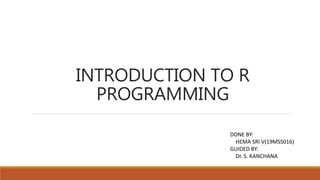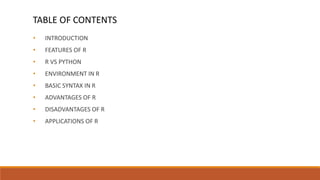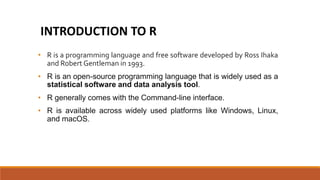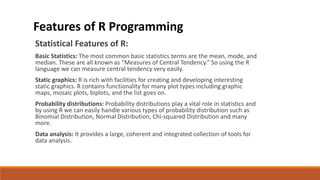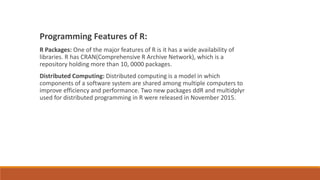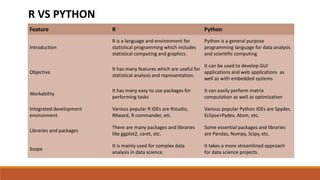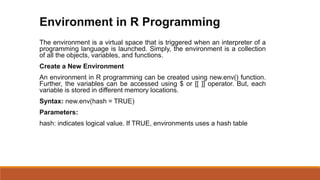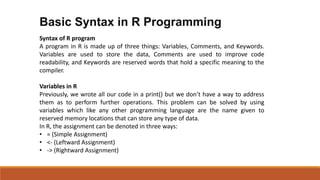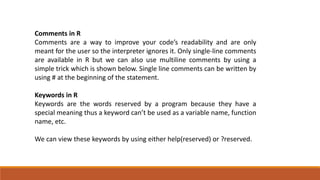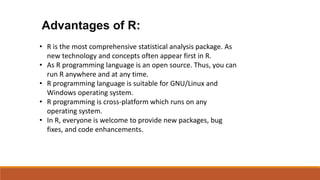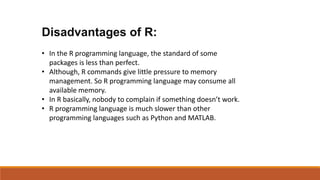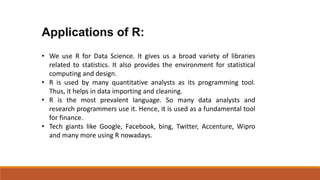1 sur 13
Publicité

### Introduction to R Programming

1. INTRODUCTION TO R PROGRAMMING DONE BY: HEMA SRI V(19MSS016) GUIDED BY: Dr. S. KANCHANA
2. • INTRODUCTION • FEATURES OF R • R VS PYTHON • ENVIRONMENT IN R • BASIC SYNTAX IN R • ADVANTAGES OF R • DISADVANTAGES OF R • APPLICATIONS OF R TABLE OF CONTENTS
3. • R is a programming language and free software developed by Ross Ihaka and Robert Gentleman in 1993. • R is an open-source programming language that is widely used as a statistical software and data analysis tool. • R generally comes with the Command-line interface. • R is available across widely used platforms like Windows, Linux, and macOS. INTRODUCTION TO R
4. Statistical Features of R: Basic Statistics: The most common basic statistics terms are the mean, mode, and median. These are all known as “Measures of Central Tendency.” So using the R language we can measure central tendency very easily. Static graphics: R is rich with facilities for creating and developing interesting static graphics. R contains functionality for many plot types including graphic maps, mosaic plots, biplots, and the list goes on. Probability distributions: Probability distributions play a vital role in statistics and by using R we can easily handle various types of probability distribution such as Binomial Distribution, Normal Distribution, Chi-squared Distribution and many more. Data analysis: It provides a large, coherent and integrated collection of tools for data analysis. Features of R Programming
5. Programming Features of R: R Packages: One of the major features of R is it has a wide availability of libraries. R has CRAN(Comprehensive R Archive Network), which is a repository holding more than 10, 0000 packages. Distributed Computing: Distributed computing is a model in which components of a software system are shared among multiple computers to improve efficiency and performance. Two new packages ddR and multidplyr used for distributed programming in R were released in November 2015.
6. Feature R Python Introduction R is a language and environment for statistical programming which includes statistical computing and graphics. Python is a general purpose programming language for data analysis and scientific computing Objective It has many features which are useful for statistical analysis and representation. It can be used to develop GUI applications and web applications as well as with embedded systems Workability It has many easy to use packages for performing tasks It can easily perform matrix computation as well as optimization Integrated development environment Various popular R IDEs are Rstudio, RKward, R commander, etc. Various popular Python IDEs are Spyder, Eclipse+Pydev, Atom, etc. Libraries and packages There are many packages and libraries like ggplot2, caret, etc. Some essential packages and libraries are Pandas, Numpy, Scipy, etc. Scope It is mainly used for complex data analysis in data science. It takes a more streamlined approach for data science projects. R VS PYTHON
7. The environment is a virtual space that is triggered when an interpreter of a programming language is launched. Simply, the environment is a collection of all the objects, variables, and functions. Create a New Environment An environment in R programming can be created using new.env() function. Further, the variables can be accessed using \$ or [[ ]] operator. But, each variable is stored in different memory locations. Syntax: new.env(hash = TRUE) Parameters: hash: indicates logical value. If TRUE, environments uses a hash table Environment in R Programming
8. Syntax of R program A program in R is made up of three things: Variables, Comments, and Keywords. Variables are used to store the data, Comments are used to improve code readability, and Keywords are reserved words that hold a specific meaning to the compiler. Variables in R Previously, we wrote all our code in a print() but we don’t have a way to address them as to perform further operations. This problem can be solved by using variables which like any other programming language are the name given to reserved memory locations that can store any type of data. In R, the assignment can be denoted in three ways: • = (Simple Assignment) • <- (Leftward Assignment) • -> (Rightward Assignment) Basic Syntax in R Programming
9. Comments in R Comments are a way to improve your code’s readability and are only meant for the user so the interpreter ignores it. Only single-line comments are available in R but we can also use multiline comments by using a simple trick which is shown below. Single line comments can be written by using # at the beginning of the statement. Keywords in R Keywords are the words reserved by a program because they have a special meaning thus a keyword can’t be used as a variable name, function name, etc. We can view these keywords by using either help(reserved) or ?reserved.
10. • R is the most comprehensive statistical analysis package. As new technology and concepts often appear first in R. • As R programming language is an open source. Thus, you can run R anywhere and at any time. • R programming language is suitable for GNU/Linux and Windows operating system. • R programming is cross-platform which runs on any operating system. • In R, everyone is welcome to provide new packages, bug fixes, and code enhancements. Advantages of R:
11. • In the R programming language, the standard of some packages is less than perfect. • Although, R commands give little pressure to memory management. So R programming language may consume all available memory. • In R basically, nobody to complain if something doesn’t work. • R programming language is much slower than other programming languages such as Python and MATLAB. Disadvantages of R:
12. • We use R for Data Science. It gives us a broad variety of libraries related to statistics. It also provides the environment for statistical computing and design. • R is used by many quantitative analysts as its programming tool. Thus, it helps in data importing and cleaning. • R is the most prevalent language. So many data analysts and research programmers use it. Hence, it is used as a fundamental tool for finance. • Tech giants like Google, Facebook, bing, Twitter, Accenture, Wipro and many more using R nowadays. Applications of R:
13. THANK YOU REFERENCE : https://www.geeksforgeeks.org/
Publicité